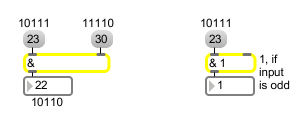# &

Bitwise intersection of two numbers

## Description

The & object performs a bit-by-bit "and" of two numbers (expressed in binary for the task). It is similar to the operator found in the C programming language.

## Arguments

Name Type Opt Description
initial-value int opt Sets an initial value to be compared with a number received in the left inlet.

## Messages

 bang In left inlet: Performs the comparison with the numbers currently stored. If there is no argument, & initially holds 0 for comparison. int input [int] In left inlet: The number is compared, in binary form, with the number in the right inlet. The output is a number composed of those bits which are 1 in both numbers. (inlet1) comparison-number [int] In right inlet: The number is stored for comparison with a number received in the left inlet. float input [float] Converted to int. set set-input [int] In left inlet: The word set followed by a number will set the input to the bitwise-and operation without causing output (a successive bang will output the result). list input [list] In left inlet: Compares the first and second numbers bit-by-bit, and outputs a number composed of those bits which are 1 in both numbers.

## Output

int: The two numbers received in the inlets are compared, one bit at a time. If a bit is 1 in both numbers, it will be 1 in the output number, otherwise it will be 0 in the output number.

## ExamplesNonzero bits shared by both numbers........ Can be used as an odd/even detector